# How to Measure and Control Multiple Wavelengths for Metrology Applications

## Introduction

The following application note describes how to use the Zurich Instruments MFLI, a 500 kHz Lock-in Amplifier, for the purpose of measuring, stabilizing, and controlling the relative distance of two interferometer paths to sub-wavelengths precision over multiple wavelengths. Through introducing a phase modulation on one of the interferometer arms, a signal is produced to show the relative displacement linearly and continuously. This signal is then handled by an internal PID controller to stabilize the relative path length to an adaptable setpoint.

## Motivation

The relative phase difference between two paths of light propagation is precisely measured by optical interferometers. They can be applied in the detection and control of changes in relative optical path length of two interferometer arms to a fraction of the light wavelength as employed in for example quantitative phase imaging .

The Michelson-Morley scheme of interferometry is shown in Figure 1, but alternate configurations such as Mach-Zehnder are also commonplace. Small relative changes of refractive index in both pathways, which can be the result of changes in air temperature or pressure, will cause large measurable signal.

One drawback of this high sensitivity is that tiny fluctuations, such as those introduced by mechanical noise or varying temperature gradients, are capable of causing tiny mirror displacements and therefore cause systematic measurement errors. Such sources of error can be helped by continuous measurement and active stabilization of the relative interferometer phase angle. But, using the relative phase angle as a direct input for a feedback system limits the control range to lower than π/2 or a quarter of wavelength, in addition to other disadvantages.

Here, a elegant technique introduced in  founded on the work in  is presented, offering these advantages:

• Working at relative phase angles that are arbitrary
• Covering an arbitrary large range of displacement
• Simple to set up and monitor
• Operation controlled manually and by computer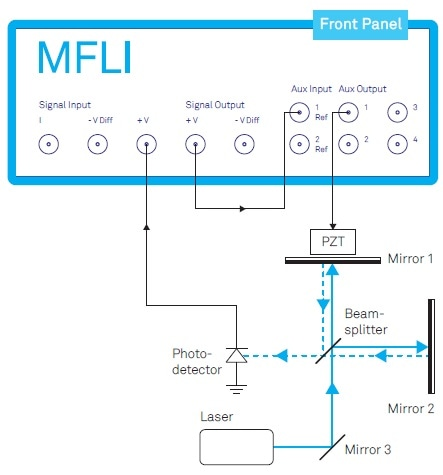Figure 1. Michelson-Morley interferometer actively stabilized by a closed-loop system using the Zurich Instruments MFLI Lock-in Amplifier. The Auxiliary Output provides both a modulating signal and a controlling offset for the piezo electric transducer (PZT). The external loop-back from the Signal Output to the Auxiliary Input is required to generate the linearized and continuous error signal for PID control (see text).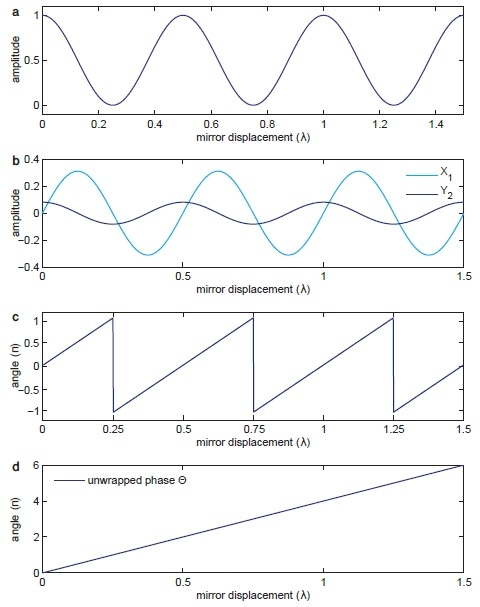Figure 2. (a) shows the sinusoidal signal detected by the photodetector over the relative displacement between mirrors 1 and 2. (b) shows the signals X1 and Y2 demodulated at Ω and 2Ω. (c) shows the phase angle between the normalized X1 and Y2 components given in Equation 5. (d) shows the unwrapped version of the same phase in (c) used by the PID controller to drive the PZT.

## Description

In the example provided in Figure 1, the light intensity I detected by the photodetector is described by Equation 1.

Equation 1: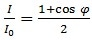In Equation 1, I0 is the intensity of the laser light at wavelength λ and the phase differences between the two arms of the interferometer is described by φ.

Figure 2(a) shows the photodetector signal described in Equation 1. It depicts two challenges to detecting the mirror displacement by the phase φ with the photodetector signal. One challenge is the discrepancy in sensitivity of the points of maximum slope and the extrema of the curve. The second challenge is to eliminate the uncertainty caused by the photodetector signal I as a non-monotone function of φ.

It is not possible to see if the displacement is continuing in the same direction or going through a turning point when reading the photodetector voltage at extrema points. Both these challenges are solvable by introducing a phase modulation to one beam path, for example by employing a sinusoidal voltage of frequency Ω to the piezoelectric transducer, which is in control of the relative path difference. Such phase modulation is described by Equation 2.

Equation 2: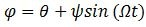In Equation 2, ψ represents the modulation depth and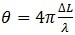is the first phase offer because of the length difference ∆L between the interferometer arms. Replacing Equation 2 for Equation 1’s φ causes a phase-modulated signal made up of higher harmonics Ω. Utilizing the Jacobi-Anger expansion, the initial and second harmonics, I1 and I2, are expressed in terms of Bessel functions J1 and J2 at the modulation depth ψ as described in Equation 3(a) and (b).

Equation 3(a):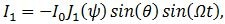Equation 3(b):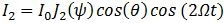Equation 4(a):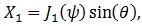Equation 4(b):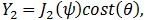In these, the components of Equation 4(a) and (b) look like the form of the initial and second derivatives of the original fringe pattern. Figure 3 depicts how the slowly-varying signal components X1 and Y2, shown in Figure 2(b), are removed from the original photodetector signal via demodulation at the frequencies Ω and 2Ω.

It is necessary that both components of X1 and Y2 contribute with precisely the same strength to linearize the response signal. This is done via adjusting the modulation depth to ψ=2.63 rad where J1(ψ) and J2 (ψ) are the same, or by presenting the correction factors J1 and J2, as seen in Figure 3. In both alternatives, the subsequent parametric plot of these two signals should portray a circle, not an ellipse. This way, the phase offset θ is acquired by Equation 5.

Equation 5: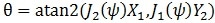It is necessary to observe that the function ‘atan2’ is utilized to decide unambiguously the phase within a range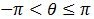from the two arguments. Figure 2(c) displays the result of this in comparison to the original photodetector signal.

A phase unwrap is carried out to extend the accessible phase range from the ‘atan2’ operation to an infinite scale as a last step to get a monotonic function of displacement between the arms. The subsequent unwrapped phase signal by the relative displacement in units of wavelengths is depicted in Figure 2(d).

This signal is now capable of being used by a PID controller to give feedback to the PZT as a offset voltage to manage the relative lengths of the paths of the interferometer and maintain the phase at exactly the defined setpoint of the PID controller. The residual error signal of the closed-loop controller then provides a direct measure of the envisioned and unintended disturbances of the setup.

A vital bit of this setup is the modulation in one of the interferometer arms. This evidently presents the limitation that the stabilization is not absolute but merely average. But, for pulsed laser studies where the stabilization is carried out with a CW laser, a proper synchronization with the repetition rate of the pulsed laser will eliminate this issue.

## Implementation

When used, the mechanical resonance frequency of Figure 1’s piezo-mirror assembly is a few ten kHz at its height, which presents an upper limit to the speed of modulation and the loop filter bandwidth. The MFLI is capable of handling modulation bandwidth of as high as 200 kHz, restrained by the Auxiliary Output bandwidth, while the bandwidth of the photodetector can go up to 5 MHz.

The maximum loop filter bandwidth is dependent on the setup properties and can go up to 50 kHz. To apply the setup depicted in Figure 3, the MFLI has to be equipped with the MF-MD Multi-demodulation and the MF-PID Quad PID/PLL Controller choices. Figure 4 depicts the technique used to apply the correction coefficients J1(ψ) and J2 (ψ) and to decide the phase between the output of two distinct demodulators.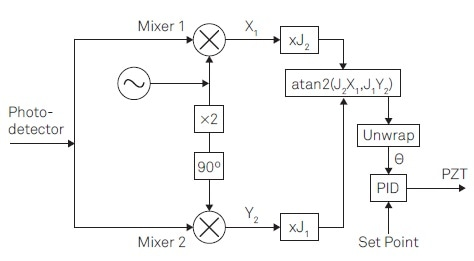Figure 3. Linear phase control for interferometer stabilization requires the following steps: (1) Provide a reference signal at Ω to apply a phase modulation. (2) Apply lock-in detection to the photodetector signal to obtain the demodulation signalsX1 and Y2 at the modulation frequency Ω and its 2nd harmonic. (3) Apply correction factors J1 and J2. (4) Determine the phase _ by atan2(J2X1; J1Y2) (5) Unwrap the phase _. (6) Apply PID controller to provide feedback.

The reference signal at frequency Ω is produced in the path of demodulator (Demod) 4 and directed through the Auxiliary Output to propel the PZT. The photodetector signal, which encompasses several harmonics of Ω as a result of the phase modulation, is received at the Signal Input and demodulated at Ω by Demod 1 to acquire X1 and 2Ω by Demod 2 to obtain Y2, as depicted in Figure 4.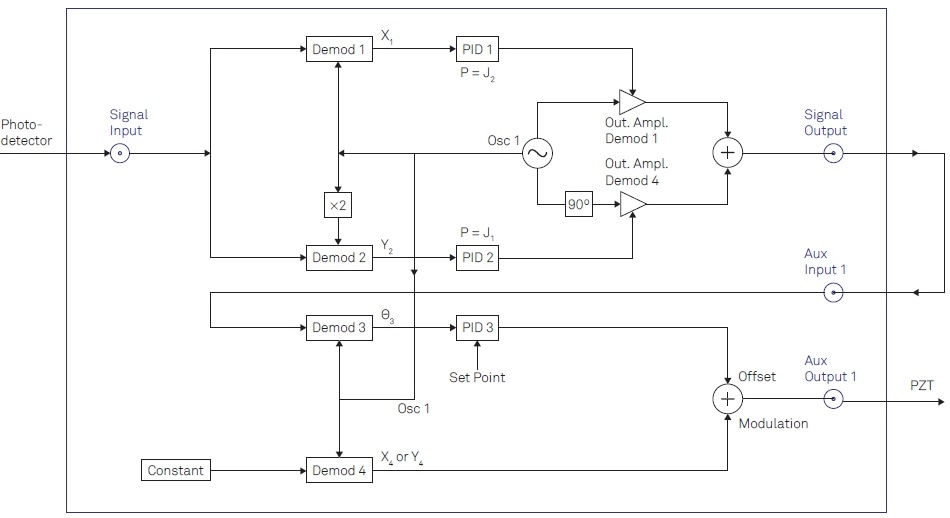Figure 4. Detailed description of the instrument configuration to be implemented using the LabOne user interface. In total, 4 demodulators (Demods) of the instruments are required. Two PID controllers are used as proportionate functions to apply the Bessel coefficients. The third PID controller is used to drive the offset signal controlling the mirror displacement.

J1(ψ) and J2 (ψ), the correction factors, are applied to the demodulated signals X1 and Y2 utilizing the PID controllers 1 and 2 in their balanced mode, meaning I and D parts are set to zero. The PID output will then modify the amplitude of a sine and cosine wave at Ω through the path of Desmod 1 and 4. The subsequent signal available at the Signal Output is expressed in Equation 6.

Equation 6: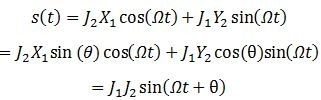Equation 6 states that the phase difference θ because of the length differences between the two arms of the interferometer is now capable of being extracted by demodulating s(t) at frequency Ω. To do this, the Signal Output is looped back to the Auxiliary Input and demodulating the signal by utilizing Demod 3, as depicted in Figure 4.

Equation 5 and Figure 2(c) show the phase extracted by Demod 3. The phase signal is subsequently used as an input for PID 3, which unwraps the phase prior to comparison of the signal to the setpoint. Once it has been passed through the PID controller, the signal is added to the reference signal in the Auxiliary Output connected to the PZT to seal the loop.

To set this up correctly, LabOne®, the control software by Zurich Instruments, offers a web-based operator interface with several powerful tools for extended analysis. Primarily, one could, for example, characterize the transfer function of the PZT-mirror assembly via recording a Bode plot using the Parametric Sweeper tool.

The frequency where the phase nears ±90° is an approximate indication of the maximum loop filter bandwidth. After this, a user could show the signals X1 and Y2 in the integrated FFT Spectrum Analyzer to verify the signal dynamics. As soon as a good estimate for the desired loop filter bandwidth is acquired, the gain parameters for PID 3 are easily capable of being derived from the PID Adviser and subsequently iteratively refined.

This whole process can in addition be automated by utilizing any of the LabOne APIs, offered for MATLAB®, LabVIEW®, Python, .NET, and C, to integrate it in an available control environment.

## Quick Modulation

Setups demanding high-speed modulators, for instance accustom-optical modulators (AOM) or electro-optical modulators (EOM), are capable of working at modulation frequencies of a few hundred MHz. This allows for elevated loop-filter bandwidths to scratch out noise components at higher frequencies. In such cases, Zurich Instruments UHFL, a 600 MHz Lock-in Amplifier, is recommended, which is also capable of being equipped with four PID controllers to employ the above scheme.

The primary difference in implementation with the UHFLI is its capability of generating the reference signal at a Signal Output instead of an Auxiliary Output. It removes the limitation of the modulation frequency to the bandwidth of the Auxiliary Output, so that the reference signal is capable of covering the entire frequency range of the instrument, in this case as high as 600 MHz.

The alternate Signal Output is utilized for the signal s(t) covering the phase information, which once more is looped back to one of the Signal Inputs to extricate its phase. The alternate Signal Input gets the photodetector signal to demodulate it at the initial and second harmonics.

The UHF-MF Multi-frequency choice is needed to freely pick several demodulators and assign them to suitable signals and adjust their frequency and phase. Three of the four PID controllers offered by the UHF-PID Quad PID/PLL Controller choice are additionally demanded to use the correction factors and to create the control signal for mirror displacement.

## Conclusion

Through the MFLI and UHFLI, Zurich Instruments offer single-instrument solutions for interferometer stabilization and control of modulation frequencies of up to 600 MHz. The scheme showed here can be rapidly and simply implemented with the LabOne operator interface which has several innate tools to characterize and monitor the setup of the study. LabOne APIs also offer full computer control.

## References

 H. Iwai, C. Fang-Yen, G. Popescu, A. Wax, K. Badizadegan, R. R. Dasari, and M. S. Feld. Quantitative phase imaging using actively stabilized phase-shifting low-coherence interferometry. Opt. Lett., 29(20):2399–2401, 2004.

 A. A. Freschi and J. Frejlich. Adjustable phase control in stabilized interferometry. Opt. Lett., 20(6):635–637, 1995.

 U. Minoni, E. Sardini, E. Gelmini, F. Docchio, and D. Marioli. Adjustable phase control in stabilized interferometry. Rev. Sci. Instrum., 62:2579, 1991.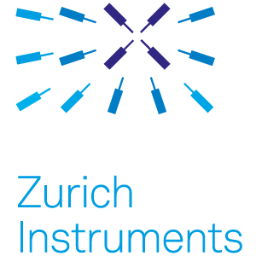This information has been sourced, reviewed and adapted from materials provided by Zurich Instruments.

## Citations

• APA

Zurich Instruments. (2019, August 23). How to Measure and Control Multiple Wavelengths for Metrology Applications. AZoM. Retrieved on October 28, 2020 from https://www.azom.com/article.aspx?ArticleID=16753.

• MLA

Zurich Instruments. "How to Measure and Control Multiple Wavelengths for Metrology Applications". AZoM. 28 October 2020. <https://www.azom.com/article.aspx?ArticleID=16753>.

• Chicago

Zurich Instruments. "How to Measure and Control Multiple Wavelengths for Metrology Applications". AZoM. https://www.azom.com/article.aspx?ArticleID=16753. (accessed October 28, 2020).

• Harvard

Zurich Instruments. 2019. How to Measure and Control Multiple Wavelengths for Metrology Applications. AZoM, viewed 28 October 2020, https://www.azom.com/article.aspx?ArticleID=16753.

## Tell Us What You Think